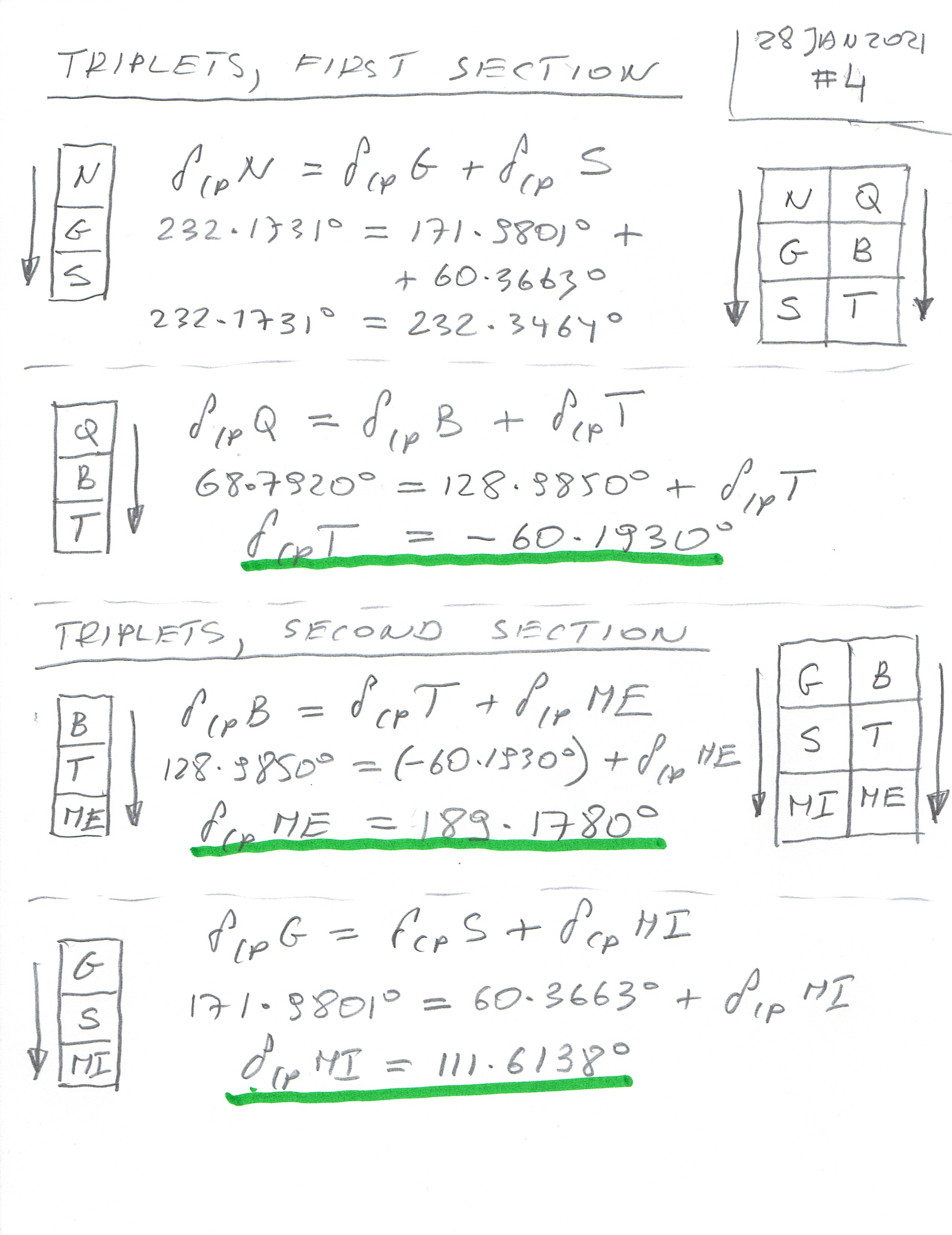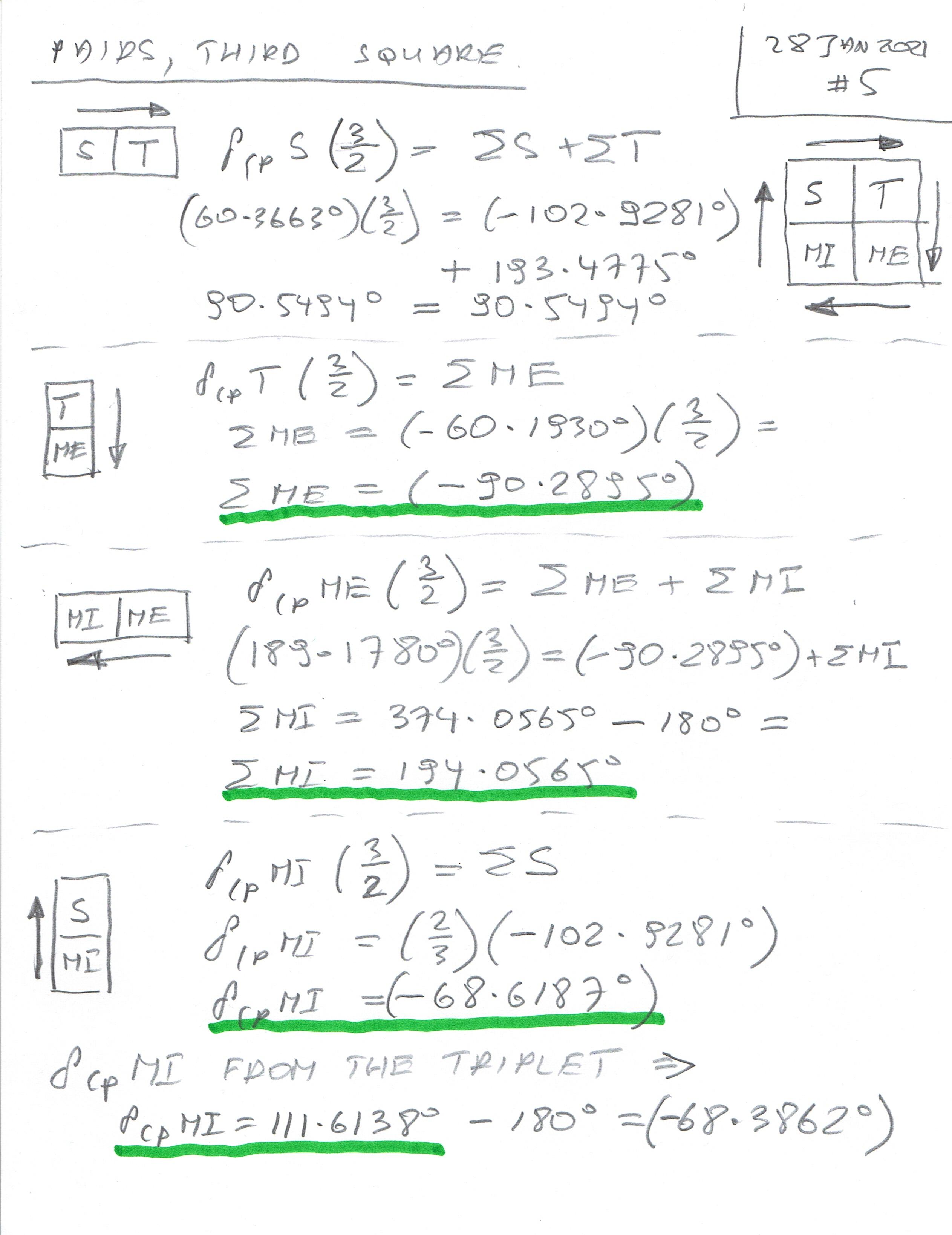Transfiguration of Our Lord; St Sixtus II (258 AD); St Felicissimus (258 AD); St Agapitus (258)

In this article, I will show how to calculate the remaining elements (Delta and Sigma Angles) for Time, Membrane Internal, and Membrane External. It is necessary to get some values from the triplets (four of them) before we can proceed to the last square with the four pairs.

The formula for triplets will be similar to that of pairs, and the last four pairs will be a test also to check if the formulas for triplets are correct (and they are!).

Triplets:

Neutrino, Graviton, Space

Quark, Boson, Time

Boson, Time, Membrane External

Graviton, Space, Membrane Internal.

Again, by analogy, the first triplet is obtained from the previous results:

Delta CP Neutrino = Delta CP Graviton + Delta CP Space

The other triplets are:

Delta CP Quark = Delta CP Boson + Delta CP Time

And from the last section we have two triplets:

Delta CP Boson = Delta CP Time + Delta CP Membrane External

and

Delta CP Graviton = Delta CP Space + Delta CP Membrane Internal

The scripts show the Triplets and the calculations of the Delta and Sigma Angles.

The last square contains also four following pairs:

Space, Time

Time, Membrane External

Membrane External, Membrane Internal

Membrane Internal, Space.

and the formulas are similar to those for the previous pairs:

Delta CP Space * (3/2) = Sigma Space + Sigma Time

Delta CP Membrane External * (3/2) = Sigma Membrane External + Sigma Membrane Internal

and

Delta CP Time * (3/2) = Sigma Membrane External

Delta CP Membrane Internal * (3/2) = Sigma Space

See the calculations and diagrams in the scripts below.

In the next article, I will calculate the Quadruplets of the elements (just to check the results obtained here and in the previous article).